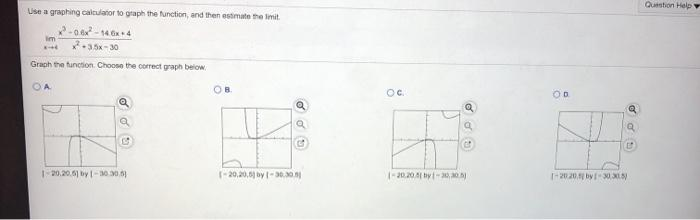### Create an Account

Already have account?

### Forgot Your Password ?

Home / Questions / Question Help Use a graphing calculator to graph the function, and then estimate the limit...

# Question Help Use a graphing calculator to graph the function, and then estimate the limit im 2.35 -30 Graph the function. Choose the correct graph below ОА OB OD a 120.20.61 by 100 1 - 20,20.0 by 130

Question Help Use a graphing calculator to graph the function, and then estimate the limit im 2.35 -30 Graph the function. Choose the correct graph below ОА OB OD a 120.20.61 by 100 1 - 20,20.0 by 1300 1 -20.20 2001 T-2020.4 by 30 31Apr 19 2021 View more View Less

#### Answer (Solved)Subscribe To Get Solution